# OR/15/015 The Fulmar rMCZ

 Lark, R M. 2015. Mapping seabed sediments of the Fulmar rMCZ. British Geological Survey Internal Report, OR/15/015.

## Data

The data used in this work were obtained by Cefas in 2012 (Ware & Meadows, 2013). A total of 65 stations were sampled by 0.1 m2 Hamon grab on a pre-planned survey grid. JNCC required that only PSA data supplied by JNCC specifically for this work should be used to produce the outputs. This is to remove compatibility issues arising from the use of PSA data originating from differing grab techniques.

The data provided are percent by mass of gravel (particles diameter > 2mm), mud (particles diameter < 0.063 mm) and sand (particles 2mm > diameter > 0.063 mm).

### Exploratory data analysis and transformation

The number of data (65) is marginal for spatial modelling (Webster and Oliver, 1992). Exploratory statistics of the 65 particle-size data are shown in Table 1 below. Note that one zero value was recorded for gravel content. As explained by Lark et al. (2012) zero values cannot be subject to the additive log-ratio transformation that is required for compositional variates such as particle-size data. We therefore imputed a small value (0.005%) for all zero observations then renormalized the values to sum to 100 for each observation. This is the same procedure used by Lark et al. (2012).

The particle-size data were then subject to an additive log-ratio (alr) transformation. This replaces a n-variate composition with n–1 transformed values, the natural logarithm of the ratio of two of the components of the composition to the third. In this case we used the gravel content as the denominator of the log-ratio so our two variables are alr-mud and alr-sand where

alr-mud = loge (mud content /gravel content), (1)
alr-sand = loge (sand content /gravel content), (2)

and mud content, gravel content and sand content are all percent by mass. Note that the choice of component to form the denominator of the log-ratio does not affect the outcome of spatial prediction by compositional cokriging (Pawlowsky-Glahn and Olea, 2004). We decided to use gravel as the denominator because it gave log-ratios of similar variability. Table 2 shows summary statistics of the alr-transformed data.

 Gravel /% Mud /% Sand /% Mean 2.51 25.55 71.94 Minimum 0.00 12.20 33.35 Maximum 34.96 54.12 85.75 Standarddeviation 6.01 7.82 10.24
 alr-mud alr-sand Mean 3.67 4.75 Minimum –1.05 0.41 Maximum 7.96 9.75 Standard deviation 1.64 1.73 Skewness –0.40 –0.26

## Spatial analysis

Before modelling the linear coregionalization, exploratory spatial analysis was undertaken. There is a pronounced cluster of observations in the south west corner of the rMCZ, and the exploratory analysis indicated that this clustering was a potential source of artefacts for spatial modelling. Whilst some close-spaced observations are desirable for the estimation of spatial models, this pronounced clustering can cause problems because of over-representation of one part of the region (e.g. Marchant et al., 2013). For this reason the cluster of points was removed leaving 52 data for spatial modelling (the analyses described in this section 2.2 of the report). All 65 data were used subsequently for mapping, as described in section 2.3. Auto-variograms and cross-variograms of the alr-transformed data were estimated from the data. The same method-of-moments estimator (MoM) was used as described by Lark et al. (2012).

The linear model of coregionalization (LMCR) was fitted to the MoM estimates of the auto- and cross-variograms by weighted least squares, as described by Lark et al. (2012). The fitted models are shown in Figure 1 below.Figure 1 Estimated variograms and cross-variograms with fitted linear model of coregionalization for alr-mud and alr-sand at Fulmar rMCZ.

The LMCRs were then compared by cross-validation, in which each observation was predicted by ordinary kriging from all remaining data. This was described in detail by Lark et al. (2012). The key diagnostic is the standardized squared prediction error, q (x), which is the square of the difference between the cross-validation prediction and the known value at location x, standardized by the ordinary kriging variance. If the kriging variance is, on average, an appropriate descriptor of the prediction uncertainty, then the mean of q (x) over all locations should be close to 1.0. As Lark et al. (2012) explain, the median is preferred as a diagnostic because of its robustness. The median of q (x) should be close to 0.455. Larger values suggest that the kriging variance is under-estimated and smaller values that it is overestimated.

 Variable alr-mud alr-sand Mean of q (x) 1.08 0.99 Median of q (x) 0.61 0.45

The cross-validation results for the auto-variograms based on MoM estimates give results very close to those expected for the correct model for alr-sand. The value of median q (x) for alr-mud is somewhat smaller than expected (although within the 95% confidence interval given the sample size). Because these results were consistent with the model fitted to the MoM estimates giving a valid account of the spatial dependence of the variables, we follow Lark (2003) in not considering robust estimators of the variograms and cross-variograms here. The LMCR based on the MoM estimates was used in further work. Its parameters are given in Table 4 . Note that there are two components to the model. The nugget component appears spatially uncorrelated at the sampling resolution used to collect the data, that is to say the variation resembles white noise because the samples are not sufficiently close to resolve the spatial structure of the factors which give rise to this variation. The second component is spatially dependent.

 Component Spatial correlation model type Distance parameter of spatial model/metres Variance or Covariance Component Auto-variog ram Cross- variogram alr-mud alr-sand 1 Nugget N.A. 1.18 1.52 1.31 2 Exponential 24646 0.68 0.87 0.70

The method used in this study is based on the assumption that the prediction errors for the transformed variables are normally distributed, with mean zero and variance given by the kriging variance. As a test of this we standardized the cross-validation errors for each variable by the square root of the ordinary kriging variance. Figure 2 shows the plot of empirical quantiles of this quantity against theoretical quantiles for a normal distribution (points), the line shows the values for a standard normal distribution of mean zero and variance 1.0. With the exception of an extreme value for alr-sand, these plots give reason for confidence in the distributional assumptions.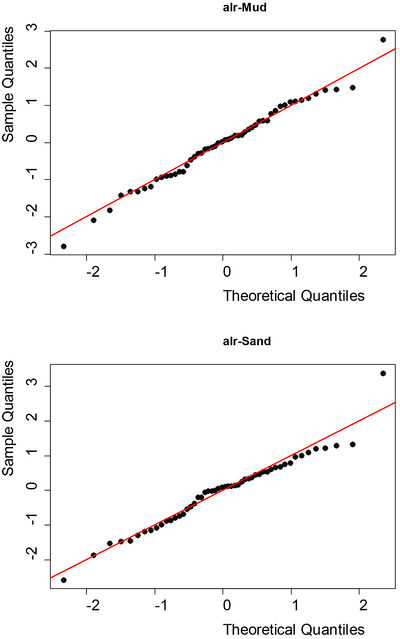Figure 2 Plot (points) of empirical quantiles of the standardized cross validation errors for alr-mud and alr-sand against the theoretical quantiles for a normal random variable. The line shows the expected values for a standard normal distribution of mean zero and variance 1.0.

## SPATIAL PREDICTIONS

Lark et al. (2012) describe the cokriging procedure used to obtain conditional expectations of the transformed variables and covariance matrices for these at target points. This procedure was undertaken to form predictions at nodes of a 250-m grid. At this stage all 65 observations in the data set were used. The simulation method used by Lark et al. (2012) was then used to generate 5000 independent realizations from the joint prediction distribution at each node. For each realization a back-transformation was undertaken to give values of gravel, mud and sand. Over all realizations the mean value of gravel, mud and sand were computed as the conditional expectation of these variables, and the 0.025 and 0.975 quantiles of the realizations were computed as confidence intervals for the predictions. It should be noted that these predictions and confidence intervals should be considered for each variable in turn.

For each realization, the EUNIS level 3 sediment texture classes (Long, 2006) were identified. At each grid node the proportion of realizations that occurred in each class is an estimate of the conditional probability of finding that class at the location. One may report the probability for each class, one may also report the class of maximum probability. The uncertainty attached to treating a site as if the class of maximum probability were the true class, can be evaluated by examining that maximum probability value, which may range from just over 1/k (where k is the number of classes) to 1.0.

The results of this analysis are held in two files.

Fulmar_predictions.dat is an ASCII format file. Each row corresponds to a node on the 250-m grid. The variables in each column of the file are shown in Table 5.

 Column Content 1 x-coordinate, UTM31N 2 y-coordinate, UTM31N 3 Estimated conditional expectation of gravel content (proportion) 4 Estimated conditional expectation of sand content (proportion) 5 Estimated conditional expectation of mud content (proportion) 6 0.025 quantile of gravel content (proportion) 7 0.975 quantile of gravel content (proportion) 8 Width of the 95% confidence interval of sand content (proportion) 9 0.025 quantile of sand content (proportion) 10 0.975 quantile of sand content (proportion) 11 Width of the 95% confidence interval of sand content (proportion) 12 0.025 quantile of mud content (proportion) 13 0.975 quantile of mud content (proportion) 14 Width of the 95% confidence interval of mud content (proportion)

Figure 3 shows the conditional expectation of gravel, sand and mud across the Fulmar rMCZ, and Figure 3 shows the 0.025 and 0.975 quantile, which define a 95% confidence interval for mud content.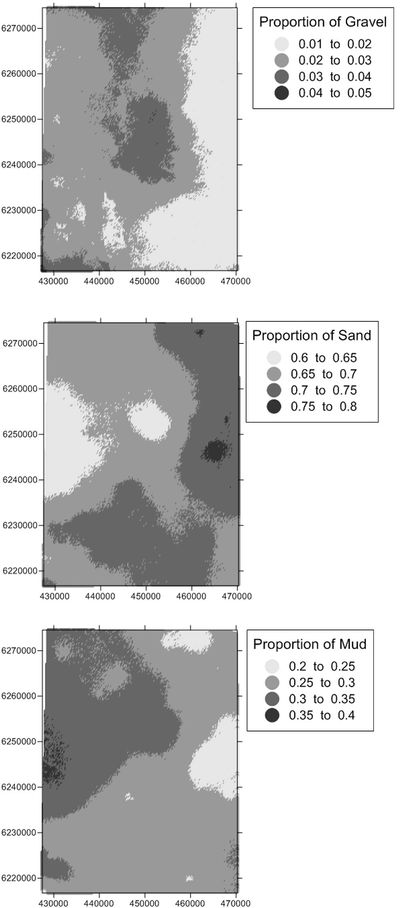Figure 3 Conditional expectation of gravel, sand and mud content (proportions) across the Fulmar rMCZ based on multiple realizations of the prediction distribution. NOTE THAT TO EMPHASIZE SPATIAL VARIATIONS EACH MAP IS ON A DIFFERENT COLOUR SCALE.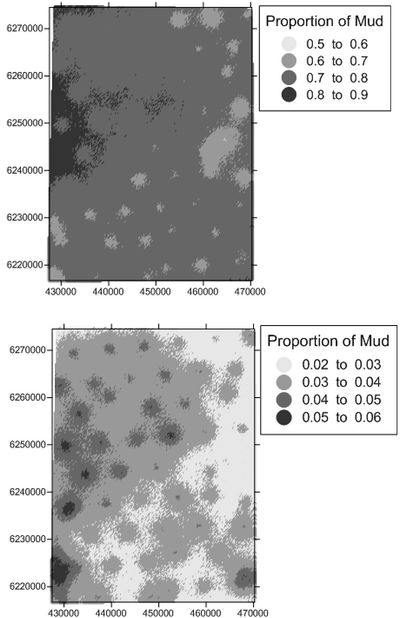Figure 4 Upper (top) and lower (bottom) bounds of the 95% confidence interval for mud content (proportion) across the Fulmar rMCZ based on multiple realizations of the prediction distribution. NOTE THAT TO EMPHASIZE SPATIAL VARIATIONS EACH MAP IS ON A DIFFERENT COLOUR SCALE.

Fulmar_classes.dat dat is an ASCII format file. Each row corresponds to a node on the 250-m grid. The variables in each column of the file are tabulated below.

 Column Content 1 x-coordinate, UTM31N 2 y-coordinate, UTM31N 3 Most probable EUNIS class:1 Coarse2 Mixed3 Mud and sandy mud4 Sand and muddy sand 4 Probability of most probable class 5 Probability of EUNIS Coarse class 6 Probability of EUNIS Mixed class 7 Probability of EUNIS Mud and sandy mud class 8 Probability of EUNIS Sand and muddy sand class 9 Entropy of the class probabilities (–1 times the expected value of the log probability over the distribution).

Figure 5 shows the most probable EUNIS class across the Fulmar rMCZ, and the probability of the most probable class. Note that the dominant classes are ‘Mud and sandy mud’ and ‘Sand and muddy sand’ Figure 6 shows the ratio of the probability of ‘Mud and sandy mud’ to ‘Sand and muddy sand’ to help elucidate the spatial pattern. Figure 7 shows the probability of each class.Figure 5 Most probable EUNIS class (top) across the Fulmar rMCZ and probability of the most probable class (bottom).

'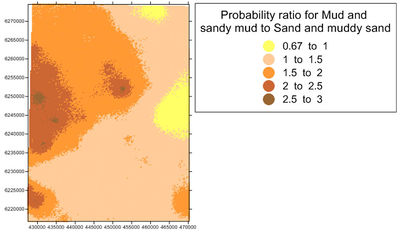Figure 6 Ratio of the probability of class Mud and sandy mud to the probability of class Sand and muddy sand.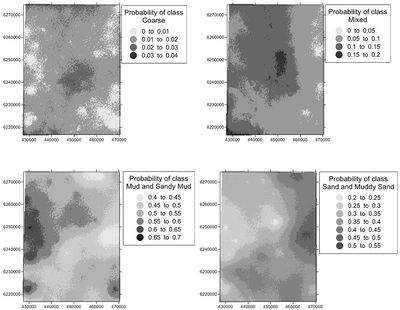Figure 7 Probability of finding each EUNIS class (top) across the Fulmar rMCZ. Note that to emphasize spatial variations each map is on a different colour scale.

The class ‘Mud and sandy mud’ is delineated as most-probable over most of the rMCZ, and the probability of this class is largest in the west of the area. However, the class ‘Sand and muddy sand’ has comparable probabilities over much of the area, and the ratio of the probability of ‘Mud and sandy mud’ to ‘Sand and muddy sand’ is nowhere larger than 3. There is therefore considerable uncertainty about the class at any location, reflected in the fact that the range of values of the probability of the most probable class does not exceed 0.7. This can also be seen in the wide confidence interval for the predicted mud content.

Uncertainty arises from the relatively sparse sampling and the limited spatial dependence of the pattern of sediment texture. Note that the variograms have a large nugget component, the apparent intercept, which represents the variability not resolved by the sampling. There might be some scope to improve the quality of these maps by incorporating the acoustic survey data that are available. In general this shows that mapping sediments in such conditions requires rather denser sampling, as well as sampling with occasional closer pairs of points across the region and not just in one corner . As noted above, the overall sample size is marginal for spatial analysis in this region, particularly after removing the one localised cluster of observations prior to variogram estimation.